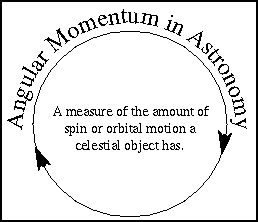The Gillespie Papers Project has been established as a private www.viewzone.com archive to discuss and evaluate the unpublished papers and notes of Dr. Rollin Gillespie. Dr. Gillespie's unique genius in the understanding of harmonics resulted in numerous inventions and advances in science. In his last work he attempts to describe the sun and planets as being a "multi-body solution to the angular momentum problem" that allows a new look at gravity and electrical attraction. Actual quotes from notes in blue blocks.Your comments and discussion is encouraged. Submit comments "here".

 Terms we will encounter...What is the Roche Radius? Roche's Limit or Roche's Radius is the distance from a planet inside which a satellite will be torn apart by tidal forces.EXAMPLE: For example, consider the rings of Saturn. All of the ring systems are inside Roche's Limit, which is the distance from a planet at which the planet's tidal force on a satellite (force that stretches apart) is equal to the satellite's self-gravity (force that holds it together). Satellites closer than Roche's limit will experience stronger tidal force, and since the self-gravity is the same regardless of distance from the planet, tidal force will dominate, and keep an object from forming, or break an already-formed object held together by self- gravity into fragments. Roche's limit, if both planet and satellite have the same density, is 2.45 planetary radii. The comparison is with the strength of self-gravity in holding the satellite together. Smaller rocks are held together by crystalline forces in the solid. Such forces are far stronger than self-gravity, and prevent them from being broken up. DETAILS: The conventional expression of Roche's Limit is that a non-cohesive satellite will break up tidally if the centre-centre distance is less than about 2.45 primary radii; the customary error is to forget the density-ratio dependence of X. What is Angular Momentum? Angular momentum measures an object's tendency to continue to spin. angular momentum = mass x velocity x distance (from point object is spinning or orbiting around)To describe how things move we often use the basic quantities of length, mass, and time. Quantities such as velocity, acceleration, force and energy are very powerful ones that help us understand how an object's position will change over time and how it will interact with other things in the universe. Momentum and its cousin angular momentum are other very powerful quantities. Ordinary (or linear) momentum is a measure of an object's tendency to move at constant speed along a straight path. Momentum depends on speed and mass. A train moving at 20 mph has more momentum than a bicyclist moving at the same speed. A car colliding at 5 mph does not cause as much damage as that same car colliding at 60 mph. For things moving in straight lines momentum is simply mass x speed. In astronomy most things move in curved paths so we generalize the idea of momentum and have angular momentum. Angular momentum measures an object's tendency to continue to spin. An "object" can be either a single body or two or more bodies acting together as a single group. angular momentum = mass x velocity x distance (from point object is spinning or orbiting around) Very often in astronomy, the object (or group of objects) we're observing has no outside forces acting on it in a way to produce torque that would disturb the angular motion of the object (or group of objects). A torque is simply a force acting along a line that is off the object's spin axis. In these cases, we have conservation of angular momentum. conservation of angular momentum ---the total amount of angular momentum does not change with time no matter how the objects interact with one another. A planet's velocity and distance from the Sun will change but the combination of speed x distance will not change unless another planet or star passes close by and provides an extra gravity force. back || Continued